Next: Driven Damped Harmonic Oscillation Up: Damped and Driven Harmonic Previous: Quality Factor

# LCR Circuits

Consider an electrical circuit consisting of an inductor, of inductance, connected in series with a capacitor, of capacitance, and a resistor, of resistance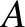. (See Figure 8.) Such a circuit is known as an LCR circuit, for obvious reasons. Suppose that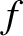is the instantaneous current flowing around the circuit. As we saw in Section 2.3, the potential drops across the inductor and the capacitor are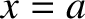and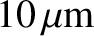, respectively. Here,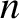is the charge on the capacitor's positive plate, and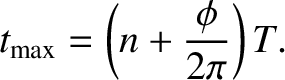. Moreover, from Ohm's law, the potential drop across the resistor is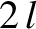(Fitzpatrick 2008). Kirchhoff's second circuital law states that the sum of the potential drops across the various components of a closed circuit loop is zero. It follows that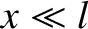(90)

Dividing by, and differentiating with respect to time, we obtain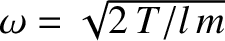(91)

where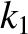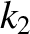(92)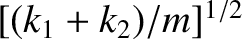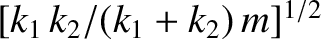(93)

Comparison with Equation (63) reveals that Equation (91) is a damped harmonic oscillator equation. Thus, provided that the resistance is not too high (i.e., provided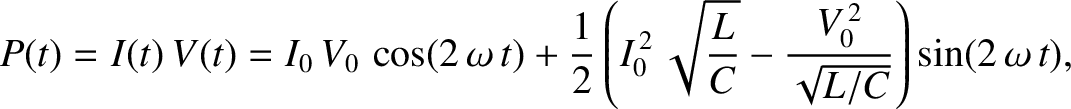, which is equivalent to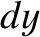), the current in the circuit executes damped harmonic oscillations of the form [cf., Equation (72)]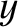(94)

whereandare constants, and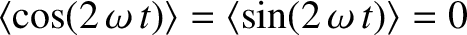. We conclude that if a small amount of resistance is introduced into an LC circuit then the characteristic oscillations in the current damp away exponentially at a rate proportional to the resistance.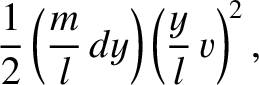Multiplying Equation (90) by, and making use of the fact that, we obtain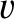(95)

which can be rearranged to give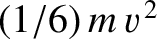(96)

where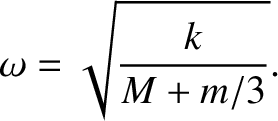(97)

Here,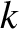is the circuit energy: that is, the sum of the energies stored in the inductor and the capacitor. Moreover, according to Equation (96), the circuit energy decays in time due to the power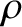dissipated via Joule heating in the resistor (Fitzpatrick 2008). Of course, the dissipated power is always positive. In other words, the circuit never gains energy from the resistor.

Finally, a comparison of Equations (89), (92), and (93) reveals that the quality factor of an LCR circuit is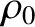(98)Next: Driven Damped Harmonic Oscillation Up: Damped and Driven Harmonic Previous: Quality Factor
Richard Fitzpatrick 2013-04-08